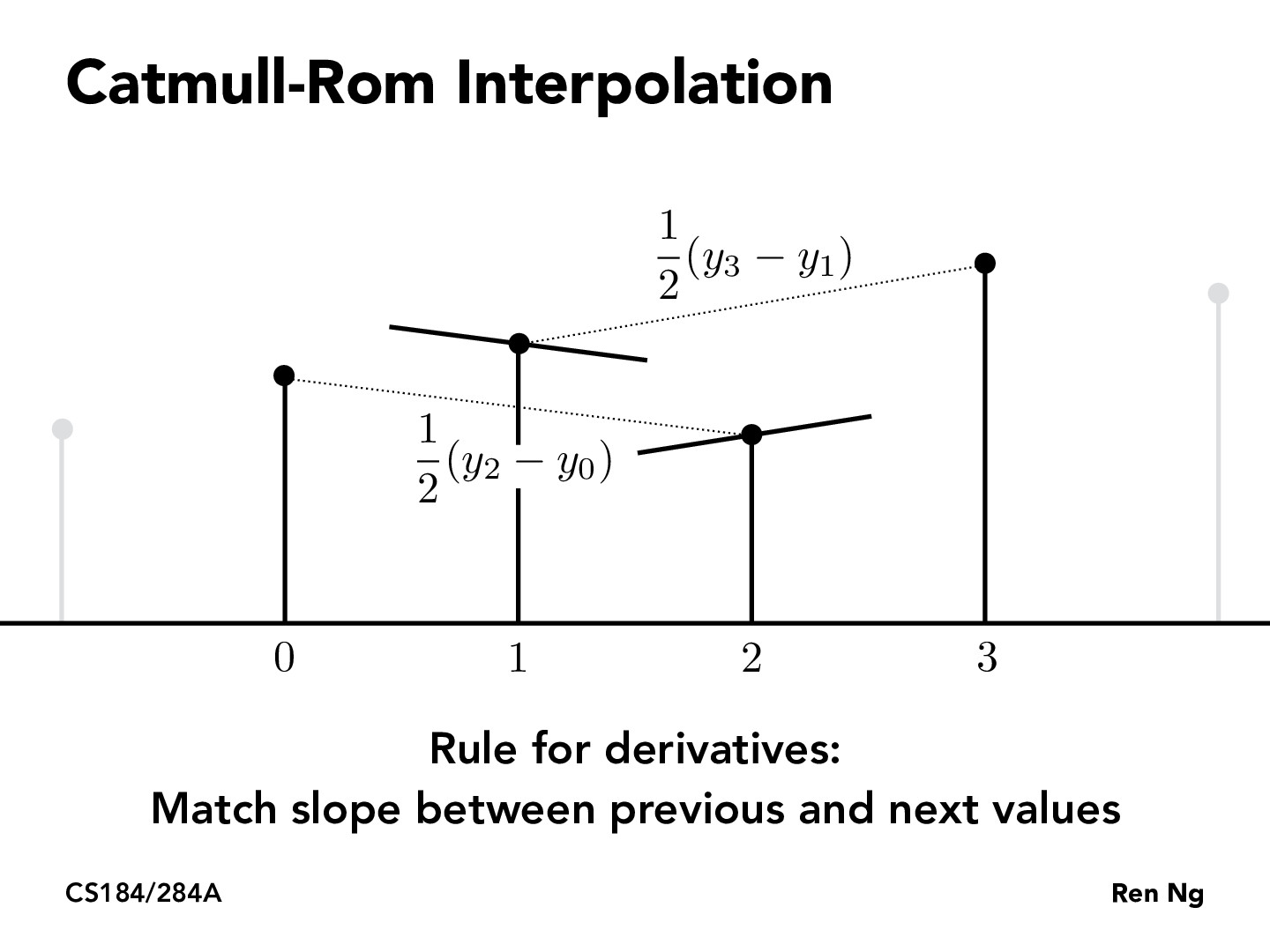Lecture 7: Geometry and Curves (44)caokevinc

Is there an interpolation method where the derivative is set to 0 for every point? It seems very arbitrary what slope we pick for each value.FLinesse

I don't think picking the slope between the previous and next points would be considered "arbitrary" as it does smooth out the curve by hugging the control points more tightly. (I think of it as picking the in-between control point to satisfy the mean value theorem)

According to Wikipedia it also states that the interpolation has the advantages of not forming self-intersections within a curve segment, as well as never forming a cusp (always continuous with well defined 1st derivative).FLinesse

Also, an interpolation method where the derivative is set to 0 for every control point does seem like an interesting idea, but how would said interpolation be used? @caokevincjcebreros1

An interpolation method with the derivative set to zero would mean the curve doesn't change(derivatives are the rate of change). I think a more interesting question would be if there is an ideal range for the derivative so that it doesn't change too sharply.andrewdotwang

When would Catmull-Rom interpolation work better than Cubic Hermite interpolation and otherwise?

You must be enrolled in the course to comment